RS Aggarwal Test: Triangles

# RS Aggarwal Test: Triangles - Class 10

Test Description

## 25 Questions MCQ Test Mathematics (Maths) Class 10 - RS Aggarwal Test: Triangles

RS Aggarwal Test: Triangles for Class 10 2023 is part of Mathematics (Maths) Class 10 preparation. The RS Aggarwal Test: Triangles questions and answers have been prepared according to the Class 10 exam syllabus.The RS Aggarwal Test: Triangles MCQs are made for Class 10 2023 Exam. Find important definitions, questions, notes, meanings, examples, exercises, MCQs and online tests for RS Aggarwal Test: Triangles below.
Solutions of RS Aggarwal Test: Triangles questions in English are available as part of our Mathematics (Maths) Class 10 for Class 10 & RS Aggarwal Test: Triangles solutions in Hindi for Mathematics (Maths) Class 10 course. Download more important topics, notes, lectures and mock test series for Class 10 Exam by signing up for free. Attempt RS Aggarwal Test: Triangles | 25 questions in 25 minutes | Mock test for Class 10 preparation | Free important questions MCQ to study Mathematics (Maths) Class 10 for Class 10 Exam | Download free PDF with solutions
 1 Crore+ students have signed up on EduRev. Have you?
RS Aggarwal Test: Triangles - Question 1

### Triangle ABC is such that AB = 3 cm, BC = 2 cm and CA = 2.5 cm. Triangle DEF is similar to ΔABC. If EF = 4 cm, then the perimeter of ΔDEF is :

Detailed Solution for RS Aggarwal Test: Triangles - Question 1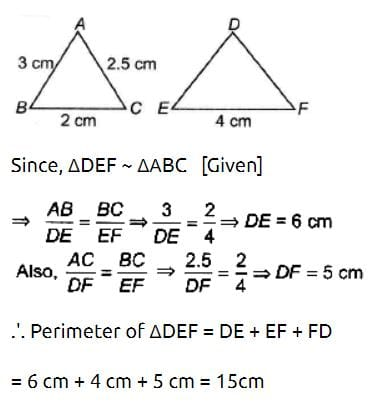RS Aggarwal Test: Triangles - Question 2

### In ΔABC, AB = 3 cm, AC = 4 cm and AD is the bisector of ∠A. Then, BD : DC is :

RS Aggarwal Test: Triangles - Question 3

### In an equilateral triangle ABC, if AD ⊥ BC, then:

Detailed Solution for RS Aggarwal Test: Triangles - Question 3

Δ ABC is an equilateral triangle

By Pythagoras theorem in triangle ABD

but BD = 1/2 BC (∵ In a triangle, the perpendicular from the vertex to the base bisects the base)

thus AB2 = AD2 + {1/2 BC}2

AB2 = AD2 + 1/4 BC2

4 AB2 = 4AD2 + BC2

4 AB2 - BC2 = 4 AD2

(as AB = BC we can subtract them )

RS Aggarwal Test: Triangles - Question 4

ABC is a triangle and DE is drawn parallel to BC cutting the other sides at D and E. If AB = 3.6 cm, AC = 2.4 cm and AD = 2.1 cm, then AE is equal to :

RS Aggarwal Test: Triangles - Question 5

The line segments joining the mid points of the sides of a triangle form four triangles each of which is :

Detailed Solution for RS Aggarwal Test: Triangles - Question 5

Given :△ABC, D, E and F are mid points of AB, BC, CA respectively.
Using mid point theorem we prove that □ADEF, □DBEF and □DECF are parallelograms. The diagonal of a parallelogram divides the parallelogram into two congruent triangles. So all triangles are congruent to each other. And each small triangle is similar to the original triangle.

RS Aggarwal Test: Triangles - Question 6

In ΔABC and ΔDEF, ∠A = 50°, ∠B = 70°, ∠C = 60°, ∠D = 60°, ∠E = 70°, ∠F = 50°, then ΔABC is similar to:

RS Aggarwal Test: Triangles - Question 7

D, E, F are the mid points of the sides BC, CA and AB respectively of ΔABC. Then ΔDEF is congruent to triangle

Detailed Solution for RS Aggarwal Test: Triangles - Question 7

The correct option is Option D.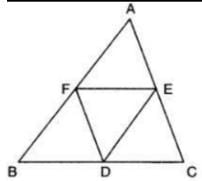Given : D, E and F are tge mid-point of the sides BC, CA and AB respectively of Δ ABC.

To prove : Δ DEF is congruent to traingle

Proof :

Since E and F are midpoints of AC and AB.

BC II FE and FE = ½ BC = BD (By mid point theorem)

BD II FE and BD = FE

Similarly, BF II DE and BF = DE

Hence, BDEF is a parallelogram (A pair of opposite sides are equal and parallel)

Similarly, we can prove that FDCE and AFDE are also parallelograms.

Now, BDEF is a parallelogram so it's diagonal FD divides it into two traingles of equal areas.

Therefore, ar(Δ BDF) = ar(Δ DEF).......... (i)

In parallelogram AFDE,

ar(Δ AFE) = ar(Δ DEF)   (EF is a diagonal)......... (ii)

In parallelogram FDCE,

ar(Δ CDE) = ar(Δ DEF)   (DE is a diagonal)...........(iii)

From (i), (ii) and (iii)

ar(Δ BDF) = ar(Δ AFE) = ar(Δ CDE) = ar(Δ DEF)..........(iv)

If area of traingles are equal then they are congruent.

Hence, Δ DEF is congruent to triangle Δ BDF = Δ AFE = Δ CDE.

RS Aggarwal Test: Triangles - Question 8

If in the triangles ABC and DEF, angle A is equal to angle E, both are equal to 40°, AB : ED = AC : EF and angle F is 65°, then angle B is :-

RS Aggarwal Test: Triangles - Question 9

In a right angled ΔABC, right angled at A, if AD ⊥ BC such that AD = p, If BC = a, CA = b and AB = c, then: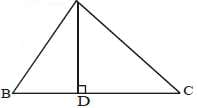Detailed Solution for RS Aggarwal Test: Triangles - Question 9

Angle B is common and Angle A=Angle D
So the triangles are similar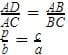a=cb/p
Now applying pythagoras theorem in
Δ ABC
H= P2+B2
BC2=AC2+AB2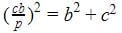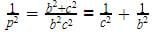RS Aggarwal Test: Triangles - Question 10

In the adjoining figure, XY is parallel to AC. If XY divides the triangle into equal parts, then the value of AX/AB  =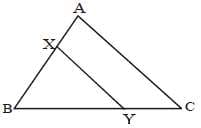RS Aggarwal Test: Triangles - Question 11

The ratio of the corresponding sides of two similar triangles is 1 : 3. The ratio of their corresponding heights is :

RS Aggarwal Test: Triangles - Question 12

The areas of two similar triangles are 49 cm2 and 64 cm2 respectively. The ratio of their corresponding sides is:

Detailed Solution for RS Aggarwal Test: Triangles - Question 12

If two triangles are similar, then the ratio of the area of both triangles is proportional to the square of the ratio of their corresponding sides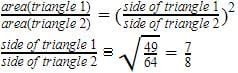RS Aggarwal Test: Triangles - Question 13

The areas of two similar triangles are 12 cm2 and 48 cm2. If the height of the smaller one is 2.1 cm, then the corresponding height of the bigger one is:

Detailed Solution for RS Aggarwal Test: Triangles - Question 13

Given,

Area of two similar triangles = 12 cm² , 48 cm² .

Area of bigger triangle = 48 cm²

Area of smaller triangle = 12 cm²

We know that, Ratio of the areas of two similar is equal to square of the ratio of corresponding heights or altitudes.

Given, Height of smaller triangle =2.1 cm.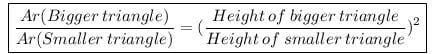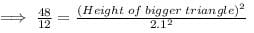4 = (Height of the triangle)² / 4.41

√(4 * 4.41) = Height of the bigger triangle

Height of the bigger triangle = 2 * 2.1 = 4.2 cm.

RS Aggarwal Test: Triangles - Question 14

In a triangle ABC, if AB, BC and AC are the three sides of the triangle, then which of the statements is necessarily true?

RS Aggarwal Test: Triangles - Question 15

The sides of a triangle are 12 cm, 8 cm and 6 cm respectively, the triangle is :

RS Aggarwal Test: Triangles - Question 16

In an equilateral triangle, the incentre, circumcentre, orthocentre and centroid are:

Detailed Solution for RS Aggarwal Test: Triangles - Question 16

The centroid is the intersection of the three medians while the incentre is the intersection of the three (internal) angle bisectors. In an equilateral triangle, each median is also an angle bisector (and vice versa), the centroid coincides with the incentre. In fact, the centroid, incentre, circumcentre and orthocentre of an equilateral triangle are coincide at the same point.

RS Aggarwal Test: Triangles - Question 17

In the adjoining figure D is the midpoint of BC of a  ΔABC. DM and DN are the perpendiculars on AB and AC respectively and DM = DN, then the ΔABC is :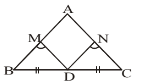RS Aggarwal Test: Triangles - Question 18

Triangle ABC is such that AB = 9 cm, BC = 6 cm, AC = 7.5 cm. Triangle ΔDEF is similar to ABC, If EF = 12 cm then DE is :

RS Aggarwal Test: Triangles - Question 19

In ΔABC, AB = 5 cm, AC = 7 cm. If AD is the angle bisector of ∠A. Then BD : CD is:

RS Aggarwal Test: Triangles - Question 20

In a ΔABC, D is the mid-point of BC and E is mid-point of AD, BF passes through E. What is the ratio of AF : FC?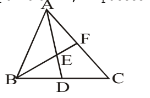Detailed Solution for RS Aggarwal Test: Triangles - Question 20

Construct DG || BF
AE = ED ( given)
EF || DG ( by construction)
a line passing through the mid point of any side of a triangle, parallel to the other side, bisects the third side
So AF = FG    ...(1)
Now in triangle CBF
BD = DC ( given)
DG||BF ( by construction)
a line passing through the mid point of any side of a triangle, parallel to the other side, bisects the third side
So, FG = GC …(2)
Now, by (1) & (2)
AF = FG = GC
AF : FC = 1:2

RS Aggarwal Test: Triangles - Question 21

In the given figure, PS is the median then ∠QPS?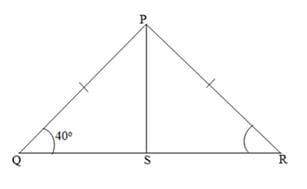Detailed Solution for RS Aggarwal Test: Triangles - Question 21

since PQ = PR

so ∠Q = ∠R = 40o

so ∠P comes out to be 100o

and since PS is a median, so it'll bisect the ∠P into two equal parts

so, ∠QPS = 50o

RS Aggarwal Test: Triangles - Question 22

The difference between altitude and base of a right angled triangle is 17 cm and its hypotenuse is 25 cm. What is the sum of the base and altitude of the triangle is ?

RS Aggarwal Test: Triangles - Question 23

If AB, BC and AC be the three sides of a triangle ABC, which one of the following is true?

RS Aggarwal Test: Triangles - Question 24

In the adjoining figure D, E and F are the mid-points of the sides BC, AC and AB respectively. ΔDEF is congruent to triangle :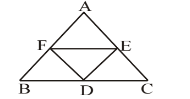Detailed Solution for RS Aggarwal Test: Triangles - Question 24

Given :△ABC, F, D and E are mid points of AB, BC, CA respectively.
Using mid point theorem we prove that □AEFD, □DBFF and □DCEF are parallelograms. The diagonal of a parallelogram divides the parallelogram into two congruent triangles. So all triangles are congruent to each other. So ΔDEF is congruent to
△AFE, △BFD and △CDE

RS Aggarwal Test: Triangles - Question 25

In the adjoining figure ∠BAC = 60° and BC = a, AC = b and AB = c, then :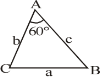Detailed Solution for RS Aggarwal Test: Triangles - Question 25

We have cosine formula as Cos A =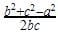So, Cos 60 =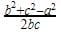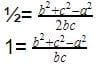b2+c2-a2=bc
a2=b2+c- bc

## Mathematics (Maths) Class 10

115 videos|478 docs|129 tests
Information about RS Aggarwal Test: Triangles Page
In this test you can find the Exam questions for RS Aggarwal Test: Triangles solved & explained in the simplest way possible. Besides giving Questions and answers for RS Aggarwal Test: Triangles, EduRev gives you an ample number of Online tests for practice

## Mathematics (Maths) Class 10

115 videos|478 docs|129 tests